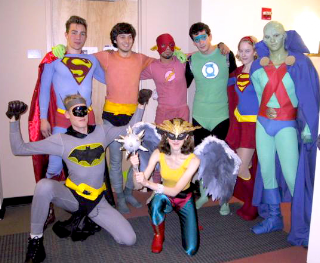## Friday, November 22, 2013

### Week 5 Maths Stratey12. Tama is making costumes for the production. Each of the costumes he makes uses  .39m2 of material. How many can he make with 33m2
85
0.39 X 10 = 3.9
0.39 X 20 = 7.8
0.39 X 40 = 15.6
0.39 X 80 = 31.2
0.39 X 82 = 31.78
0.39 X 83 = 32.17
0.39 X 84 = 32.56

0.39 X 85 = 32.95